SSC CGL Previous Year Questions: Number System and HCF & LCM- 3

# SSC CGL Previous Year Questions: Number System and HCF & LCM- 3 - SSC CGL

Test Description

## 30 Questions MCQ Test SSC CGL (Tier - 1) - Previous Year Papers (Topic Wise) - SSC CGL Previous Year Questions: Number System and HCF & LCM- 3

SSC CGL Previous Year Questions: Number System and HCF & LCM- 3 for SSC CGL 2023 is part of SSC CGL (Tier - 1) - Previous Year Papers (Topic Wise) preparation. The SSC CGL Previous Year Questions: Number System and HCF & LCM- 3 questions and answers have been prepared according to the SSC CGL exam syllabus.The SSC CGL Previous Year Questions: Number System and HCF & LCM- 3 MCQs are made for SSC CGL 2023 Exam. Find important definitions, questions, notes, meanings, examples, exercises, MCQs and online tests for SSC CGL Previous Year Questions: Number System and HCF & LCM- 3 below.
Solutions of SSC CGL Previous Year Questions: Number System and HCF & LCM- 3 questions in English are available as part of our SSC CGL (Tier - 1) - Previous Year Papers (Topic Wise) for SSC CGL & SSC CGL Previous Year Questions: Number System and HCF & LCM- 3 solutions in Hindi for SSC CGL (Tier - 1) - Previous Year Papers (Topic Wise) course. Download more important topics, notes, lectures and mock test series for SSC CGL Exam by signing up for free. Attempt SSC CGL Previous Year Questions: Number System and HCF & LCM- 3 | 30 questions in 30 minutes | Mock test for SSC CGL preparation | Free important questions MCQ to study SSC CGL (Tier - 1) - Previous Year Papers (Topic Wise) for SSC CGL Exam | Download free PDF with solutions
 1 Crore+ students have signed up on EduRev. Have you?
SSC CGL Previous Year Questions: Number System and HCF & LCM- 3 - Question 1

### A three-digit number 4a3 is added to an other three-digit number 984 to give the four digit number 13b7 which is divisible by 11. Then the value of (a + b) is:       (SSC CGL 1st Sit. 2012)

Detailed Solution for SSC CGL Previous Year Questions: Number System and HCF & LCM- 3 - Question 1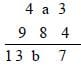∵ 13b7 is exactly divisible by 11.
∴ b = 9 ∴ a = 1
∴ a + b = 9 + 1 = 10

SSC CGL Previous Year Questions: Number System and HCF & LCM- 3 - Question 2

### The least number which when divided by 35, 45, 55 leaves the remainder 18, 28, 38 respectively is       (SSC CGL 1st Sit. 2012)

Detailed Solution for SSC CGL Previous Year Questions: Number System and HCF & LCM- 3 - Question 2

35 – 18 = 17
45 – 28 = 17
55 – 38 = 17
i.e., difference between the divisor and correseponding remainder is same.
LCM of 35, 45 and 55 = 3465
∴ Required number
= 3465 – 17 = 3448

SSC CGL Previous Year Questions: Number System and HCF & LCM- 3 - Question 3

### The number nearest to 75070 which is divisible by 65, is       (SSC CGL 1st Sit. 2012)

Detailed Solution for SSC CGL Previous Year Questions: Number System and HCF & LCM- 3 - Question 3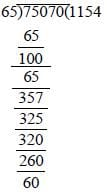∴ Required number
= 75070 + (65 – 60) = 75075

SSC CGL Previous Year Questions: Number System and HCF & LCM- 3 - Question 4

There are 4 terms in an A.P. such that the sum of two means is 110 and product of their extremes is 2125. The 3rd term is           (SSC Sub. Ins. 2012)

Detailed Solution for SSC CGL Previous Year Questions: Number System and HCF & LCM- 3 - Question 4

Let the 4 terms in A.P are a – 3d, a – d, a + d, a + 3d
According to question
a – d + a + d = 110 ... (1)
(a – 3d) (a + 3d) = 2125 ... (2)
From equation (1)
a – d + a + d = 110
2a = 110 ⇒ a = 55
From equation (2)
(a – 3d) (a + 3d) = 2125
⇒ a2 – 9d2 = 2125
⇒ (55)2 – 9d2 = 2125
⇒ 3025 – 9d2 = 2125
⇒ 900 = 9d2 ⇒ d2 = 100 ⇒ d = 10
∴ a = 55, d = + 10
series would be :
25, 45, 65, 85
IIIrd term would be 65.

SSC CGL Previous Year Questions: Number System and HCF & LCM- 3 - Question 5

The greatest number that can divide 140, 176, 264 leaving remainders of 4, 6, and 9 respectively is       (SSC Sub. Ins. 2012)

Detailed Solution for SSC CGL Previous Year Questions: Number System and HCF & LCM- 3 - Question 5

Required number = H.C.F of (140 – 4), (176 – 6) and (264 – 9) = H.C.F. of 136, 170 and 255.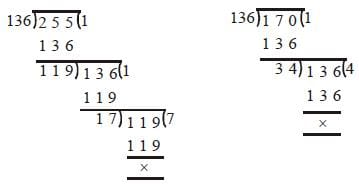∴ Required number = 17
"Alternate Method:
Here divisible terms are 140 – 4 = 136, 176 – 6 = 170
and 264 – 9 = 255
Now, difference between these numbers
170 – 136 = 34
225 – 170 = 85
H.C.F of difference = 17
Hence required number = 17."

SSC CGL Previous Year Questions: Number System and HCF & LCM- 3 - Question 6

Four runners started running simultaneously from a point on a circular track. They took 200 seconds, 300 seconds, 360 seconds and 450 seconds to complete one round. After how much time they meet at the starting point for the first time?       (SSC CGL 2nd Sit. 2011)

Detailed Solution for SSC CGL Previous Year Questions: Number System and HCF & LCM- 3 - Question 6

Required time = LCM of 200, 300, 360 and 450 seconds = 1800 seconds.

SSC CGL Previous Year Questions: Number System and HCF & LCM- 3 - Question 7

When 'n' is divisible by 5 the remainder is 2. What is the remainder when n2 is divided by 5?       (SSC CGL 2nd Sit. 2011)

Detailed Solution for SSC CGL Previous Year Questions: Number System and HCF & LCM- 3 - Question 7

Required remainder = Remainder obtained by dividing 22 by 5.
Remainder = 4

SSC CGL Previous Year Questions: Number System and HCF & LCM- 3 - Question 8

L.C.M. of two numbers is 120 and their H.C.F. is 10. Which of the following can be the sum of those two numbers?         (SSC CGL 2nd Sit. 2011)

Detailed Solution for SSC CGL Previous Year Questions: Number System and HCF & LCM- 3 - Question 8

Let the numbers be 10x and 10y where x and y are prime to each other.
∴ LCM = 10 xy
⇒ 10xy = 120 ⇒ xy = 12
Posssible pairs = (3, 4) or (1, 12)
∴ Sum of the numbers = 30 + 40 = 70
"Alternate Method:
If two different numbers are in form of ax and bx
H.C.F of these numbers is x and L.C.M of these numbers is abx
Now a and b are co-prime terms in L.C.M.
10 × a × b = 120
a × b = 12 → 1 × 12
2 × 6 this is not a pair of co prime terms.
3 × 4"

SSC CGL Previous Year Questions: Number System and HCF & LCM- 3 - Question 9

Which one of the following will completely divide 571 + 572 + 573?      (SSC CGL 2nd Sit. 2011)

Detailed Solution for SSC CGL Previous Year Questions: Number System and HCF & LCM- 3 - Question 9

571 + 572 + 573
= 571 (1 + 5 + 52) = 570 × 5 × 31
= 571 × 155 which is exactly divisible by 155.

SSC CGL Previous Year Questions: Number System and HCF & LCM- 3 - Question 10

If a * b = ab, then the value of 5 * 3 is        (SSC CGL 2nd Sit. 2011)

Detailed Solution for SSC CGL Previous Year Questions: Number System and HCF & LCM- 3 - Question 10

a * b = ab
∴ 5 * 3 = 53 = 5 × 5 × 5 = 125

SSC CGL Previous Year Questions: Number System and HCF & LCM- 3 - Question 11

The unit digit in the sum of (124)372 + (124)373 is       (SSC CGL 2nd Sit. 2011)

Detailed Solution for SSC CGL Previous Year Questions: Number System and HCF & LCM- 3 - Question 11

41 = 4; 42 = 16; 43 = 64; 44 = 256; 45 = 1024
Remainder on dividing 372 by 4 = 0
Remainder on dividing 373 by 4 = 1
∴ Required unit digit
= Unit’s digit of the sum = 6 + 4 = 0

SSC CGL Previous Year Questions: Number System and HCF & LCM- 3 - Question 12

If 17200 is divided by 18, the remainder is        (SSC CGL 2nd Sit. 2011)

Detailed Solution for SSC CGL Previous Year Questions: Number System and HCF & LCM- 3 - Question 12

Remainder when (x – 1)n is divided by x is (–1)n
∴ (17)200 = (18 – 1)200
∴ Remainder = (–1)200 = 1
"Alternate Method:
an – bn is completely divisible by a + b. If n is an even number in the case of 17200 ÷ 18 (17200 - 1200) is completely divisible by 17 + 1 = 18
Here, 1 is remainder.
Or in other words if an is divided by a + 1 and n is even number then it always left 1 as remainder."

SSC CGL Previous Year Questions: Number System and HCF & LCM- 3 - Question 13

The least number, which is to be added to the greatest number of 4 digits so that the sum may be divisible by 345, is        (SSC CGL 2nd Sit. 2011)

Detailed Solution for SSC CGL Previous Year Questions: Number System and HCF & LCM- 3 - Question 13

The largest 4-digit number = 9999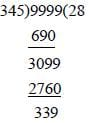∴ Required number = 345 – 339 = 6

SSC CGL Previous Year Questions: Number System and HCF & LCM- 3 - Question 14

The traffic lights at three different road crossings change after 24 seconds, 36 seconds and 54 seconds respectively. If they all change simultaneously at 10 : 15 :00 AM, then at what time will they again change simultaneously?        (SSC CGL 1st Sit. 2011)

Detailed Solution for SSC CGL Previous Year Questions: Number System and HCF & LCM- 3 - Question 14

LCM of 24, 36 and 54 seconds
= 216 seconds = 3 minutes 36 seconds
∴ Required time = 10 : 15 : 00 + 3 minutes 36 seconds = 10 : 18 : 36 a.m.

SSC CGL Previous Year Questions: Number System and HCF & LCM- 3 - Question 15

A number when divided by 49 leaves 32 as remainder. This number when divided by 7 will have the remainder as         (SSC CGL 1st Sit. 2011)

Detailed Solution for SSC CGL Previous Year Questions: Number System and HCF & LCM- 3 - Question 15

Here, the first divisor i.e. 49 is multiple of second divisor i.e. 7.
∴ Required remainder = Remainder obtained on dividing 32 by 7 = 4

SSC CGL Previous Year Questions: Number System and HCF & LCM- 3 - Question 16

The L.C.M. of three different numbers is 120. Which of the following cannot be their H.C.F.?       (SSC CGL 1st Sit. 2011)

Detailed Solution for SSC CGL Previous Year Questions: Number System and HCF & LCM- 3 - Question 16

HCF must be a factor of LCM from option 35 is not factor of 120.

SSC CGL Previous Year Questions: Number System and HCF & LCM- 3 - Question 17

If x * y = (x + 3)2 (y –1), then the value of 5 * 4 is      (SSC CGL 1st Sit. 2011)

Detailed Solution for SSC CGL Previous Year Questions: Number System and HCF & LCM- 3 - Question 17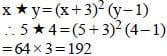SSC CGL Previous Year Questions: Number System and HCF & LCM- 3 - Question 18

The last digit of (1001)2008 + 1002 is        (SSC CGL 1st Sit. 2011)

Detailed Solution for SSC CGL Previous Year Questions: Number System and HCF & LCM- 3 - Question 18

Last digit of (1001)2008 + 1002 = 1 + 2 = 3

SSC CGL Previous Year Questions: Number System and HCF & LCM- 3 - Question 19

The remainder when 321 is divided by 5 is        (SSC CGL 1st Sit. 2011)

Detailed Solution for SSC CGL Previous Year Questions: Number System and HCF & LCM- 3 - Question 19

31 = 3; 32 = 9; 33 = 27; 35 = 81; 35 = 243
i.e. unit’s digit is repeated after index 4.
Remainder after dividing 21 by 4 = 1
∴ Unit’s digit in the expansion of (3)21 = 3
∴ Remainder after dividing by 5 = 3

SSC CGL Previous Year Questions: Number System and HCF & LCM- 3 - Question 20

How many perfect squares lie between 120 and 300 ?       (SSC CGL 2nd Sit. 2010)

Detailed Solution for SSC CGL Previous Year Questions: Number System and HCF & LCM- 3 - Question 20

112 = 121, 122 = 144, 132 = 169, 142 = 196
152 = 225, 162 = 256, 172 = 289
Square no above 120 = 121 of 11
Square no below 300 = 289 of 17
Total 11, 12, 13, 14, 15, 16, 17, i.e. 7 no.
"Alternate Method:
First square number above 120 is 121
112 > 120 and 182 > 300
Hence, required number of squares between 120 to 300
= 18 – 11 = 7"

SSC CGL Previous Year Questions: Number System and HCF & LCM- 3 - Question 21

If 'n' be any natural number, then by which largest number (n3 – n) is always divisible?        (SSC CGL 2nd Sit. 2010)

Detailed Solution for SSC CGL Previous Year Questions: Number System and HCF & LCM- 3 - Question 21

n3 – n = (n2 – 1)
⇒ n (n +1) (n – 1)
For n = 2, n3 – n = 6
23 – 2 = 6
i.e. n3 – n is always divisible by 6.

SSC CGL Previous Year Questions: Number System and HCF & LCM- 3 - Question 22

The sum of two numbers is 36 and their H.C.F and L.C.M. are 3 and 105 respectively. The sum of the reciprocals of two numbers is      (SSC CGL 2nd Sit. 2010)

Detailed Solution for SSC CGL Previous Year Questions: Number System and HCF & LCM- 3 - Question 22

Let the numbers be 3x and 3y.
∴ 3x + 3y = 36
⇒ x + y = 12 ...(i)
and 3xy = 105 ...(ii)
Dividing equation (i) by (ii), we have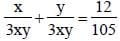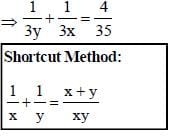SSC CGL Previous Year Questions: Number System and HCF & LCM- 3 - Question 23

The H.C.F. and L.C.M. of two numbers are 12 and 336 respectively. If one of the numbers is 84, the other is       (SSC CGL 2nd Sit. 2010)

Detailed Solution for SSC CGL Previous Year Questions: Number System and HCF & LCM- 3 - Question 23

First number × second number
= HCF × LCM
⇒ 84 × second number = 12 × 336
∴ Second number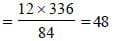p × q = HCF × LCM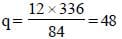SSC CGL Previous Year Questions: Number System and HCF & LCM- 3 - Question 24

A 4–digit number is formed by repeating a 2–digit number such as 1515, 3737, etc. Any number of this form is exactly divisible by       (SSC CGL 2nd Sit. 2010)

Detailed Solution for SSC CGL Previous Year Questions: Number System and HCF & LCM- 3 - Question 24

xyxy = xy × 100 + xy
= xy (100 + 1) = 101 × xy
Hence, the number is exactly divisible by 101.

SSC CGL Previous Year Questions: Number System and HCF & LCM- 3 - Question 25

A number, when divided by 136, leaves remainder 36. If the same number is divided by 17, the remainder will be         (SSC CGL 2nd Sit. 2010)

Detailed Solution for SSC CGL Previous Year Questions: Number System and HCF & LCM- 3 - Question 25

If the first divisor be a multiple of the second divisor, then required remainder = remainder obtained by dividing the first remainder (36) by the second divisor
(17) = 2
∵ 17 is a factor of 136
∴ Remainder when 36 is divided by 17 = 2

SSC CGL Previous Year Questions: Number System and HCF & LCM- 3 - Question 26

The sixth term of the sequence 2, 6, 11, 17, ..... is          (SSC CGL 1st Sit. 2010)

Detailed Solution for SSC CGL Previous Year Questions: Number System and HCF & LCM- 3 - Question 26

2 + 4 = 6
6 + 5 = 11
11 + 6 = 17
17 + 7 = 24
24 + 8 = 32

SSC CGL Previous Year Questions: Number System and HCF & LCM- 3 - Question 27

Two numbers are in the ratio 3 : 4. Their L.C.M. is 84. The greater number is        (SSC CGL 1st Sit. 2010)

Detailed Solution for SSC CGL Previous Year Questions: Number System and HCF & LCM- 3 - Question 27

Let the numbers be 3x and 4x.
∴ Their LCM = 12x
∴ 12x = 84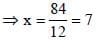∴ Larger number
= 4x = 4 × 7 = 28

SSC CGL Previous Year Questions: Number System and HCF & LCM- 3 - Question 28

A number, when divided by 114, leaves remainder 21. If the same number is divided by 19, then the remainder will be        (SSC CGL 1st Sit. 2010)

Detailed Solution for SSC CGL Previous Year Questions: Number System and HCF & LCM- 3 - Question 28

If the first divisor is a multiple of second divisor.
Then, remainder by the second divisor.
∴ Remainder = 21 ÷ 19 = 2

SSC CGL Previous Year Questions: Number System and HCF & LCM- 3 - Question 29

The ninth term of the sequence 0, 3, 8, 15, 24, 35, .... is       (SSC CGL 1st Sit. 2010)

Detailed Solution for SSC CGL Previous Year Questions: Number System and HCF & LCM- 3 - Question 29

0 + 3 = 3
3 + 5 = 8
8 + 7 = 15
15 + 9 = 24
24 + 11 = 35
35 + 13 = 48
48 + 15 = 63
63 + 17 = 80

SSC CGL Previous Year Questions: Number System and HCF & LCM- 3 - Question 30

The greatest number, which when subtracted from 5834, gives a number exactly divisible by each of 20, 28, 32 and 35, is       (SSC CGL 1st Sit. 2010)

Detailed Solution for SSC CGL Previous Year Questions: Number System and HCF & LCM- 3 - Question 30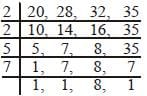∴ LCM = 2 × 2 × 5 × 7 × 8 = 1120
∴ Required number = 5834 – 1120 = 4714

## SSC CGL (Tier - 1) - Previous Year Papers (Topic Wise)

250 tests
Information about SSC CGL Previous Year Questions: Number System and HCF & LCM- 3 Page
In this test you can find the Exam questions for SSC CGL Previous Year Questions: Number System and HCF & LCM- 3 solved & explained in the simplest way possible. Besides giving Questions and answers for SSC CGL Previous Year Questions: Number System and HCF & LCM- 3, EduRev gives you an ample number of Online tests for practice

250 tests